## Python Tutorials: Regression and Classification in Python

##### Python Tutorials: In this part of Learning Python we Cover Difference Between Classification and Regression in Machine Learning
Written by |16-Oct-2020 | 0 Comments | 924 Views

Nonlinear Classification in SVM by KKT:

Karush Kuhn Tucker (KKT) is a first-order necessary condition for a solution in nonlinear programming to be optimally provided that some regularity conditions are satisfied.

KKT satisfying conditions are as follows: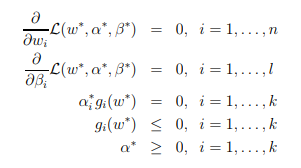Use Case of SVM:

SVM is used in a large variety of datasets. Following are some examples of daily life applications of SVM:

• Face Recognition: SVM is a more accurate and reliable classifier when it comes to face recognition. It classifies 1 for faces and -1 for nonface. Then it extracts the feature from each pixel as face or nonface. The famous python library for face recognition uses SVM for face classification.

• Hand Writing Recognition:  It is another example of in which SVM is used. Tesseract OCR and pytesseract use HOG along with SVM for classification.

• Geo and Environmental Science: We use SVMs for geo (spatial) and spatiotemporal environmental data analysis and modeling series.

SVM Optimization Technique using Kernel:

Suppose we are given an arbitrary dataset, we typically don't know how our dataset looks. Suppose e have a dataset that cannot be separable linearly, then the predicting model will fail to generate accurate results. In those particular situations, SVM kernel tricks are used for nonlinear classification of the dataset.

SVM kernel tricks are complicated and tricky and depend upon the type of dataset we are using.

SVM kernel Functions:

The support vector machine algorithm uses some functions that may vary according to the situation and the type of data set. These functions are called kernels. The functionality of a kernel is to feed the data set into and then separate the data according to the range of Kernel. We can use a different kind of Kernel in different scenarios. There is a different kind of kernels available, for example:

• Sigmoid
• Linear Kernel
• Nonlinear Kernel
• Polynomial Kernel

In industry, the most commonly used Kernel is RBF due to its extraordinary performance. It can make all the possible cuts with efficiency.

The kernel function returns the data that lies inside the cuts or between two points, which 0 and 1 in the case of sigmoid and -1,0 and 1 in the case of the hyperbolic tangent kernel.

Kernels and Similarity: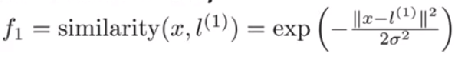In the above-given equation, f1 is the new feature that is being derived from the feature x, and l is the landmarks that are at the close distance of feature x.

|| x-l ||^2 is the euclidian distance between the feature x and landmark l.

The equation is of a Gaussian kernel of support vector machine.

If x approximately equals to l, then:

f1≈exp-(02)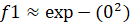/ 2sigma^2 which approximately equals to 1

if x is far from l than:

f1= exp (- (large number) ^2 / 2sigma^2) which is approximately equal to 0

Examples of SVM Kernels:

Polynomial Kernel:

In Artificial Intelligence, the polynomial piece is a bit capacity usually utilized with help vector machines (SVMs) and other kernelized models, that speaks to the similitude of vectors (preparing tests) in a component space over polynomials of the first factors, permitting learning of non-direct models.

Instinctively, the polynomial bit looks not just at the given highlights of information tests to decide their closeness, yet also blends of these. With regards to relapse investigation, such mixes are known as connection highlights.

It is popular in image processing and NLP. Mathematically it is represented as: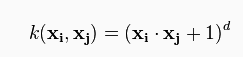where d is the degree of polynomials.

Linear SVM is a parametric model. However, an RBF kernel SVM isn't, and the difficulty of the following grows with the size of the training set. An RBF kernel SVM is more expensive to train, but you also have to retain the kernel matrix around, and it predicts into this "infinite" higher dimensional space where the data becomes linearly separable is largely expensive as well during prediction. Furthermore, you have more hyperparameters to tune, so the model selection is more expensive as well! Moreover, finally, it's much easier to overfit a complex model!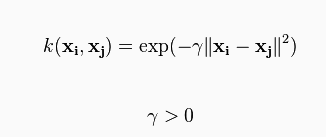Sometime parametrized using: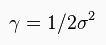Laplace RBF Kernel:

It is a type of Kernel which we preferred when there is no or less knowledge of data

Equation is: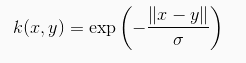where x – y is the distance from feature to landmarks

Hyperbolic Tangent Kernel:

A hyperbolic tangent kernel is based on the phenomenon of the hyperbolic tangent, which took the dot product with fixed linear scaling. This Kernel is also used extensively in the neural network as an activation function. The equation is as follows:

kernel(v1,v2) = tanh(p1 * v1 * v2 + p0)

where v is the vectors and p is the parameters in this equation

1. Kernel:

The sigmoid Kernel returns two values, 0 and 1, so for using sigmoid Kernel, there must be only two classes to classify 0 or 1; more than two classes will give the worst prediction.

The sigmoid Kernel is used in neural networking as an activation. The equation is as follows: